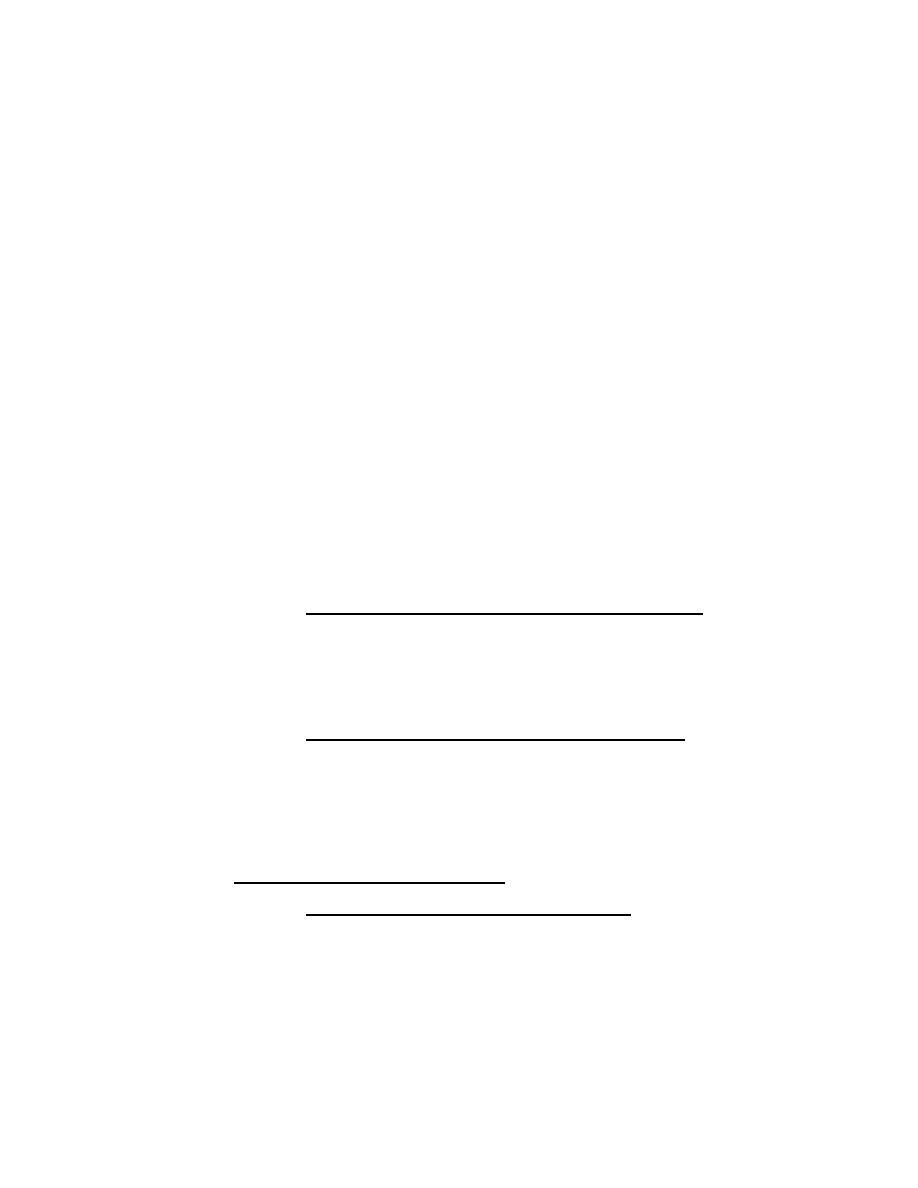Custom SearchMIL-HDBK-1013/1A
provide higher penetration times than those constructed of wood or metal at
roughly comparable costs.
Table 42
Existing Roof/Floor Concrete Construction
+))))))))))))))))))))))))))))0))))))))))))))))))))))))))))))))))),
*
*
*
Maximum Penetration Times
*
Building Component (b)  *
*
(minutes) Achievable
*
/)))))))))0))))))))))))))0))))))))))1
*
*
*
*  High
*
Low
Medium
*
/)))))))))3))))))))))))))3))))))))))1
*
*
(a)  *
*
*
Concrete Roofs
7.5
35
*
*
*
*
*
*
*
*
*
*
Concrete Floors
*
*
(a)  *
*
*
o Downward attack
7.5
35
*
*
(a)  *
*
*
o Upward attack
(2)
45
.))))))))))))))))))))))))))))2)))))))))2))))))))))))))2))))))))))-
(a)
It is not practical for threats to attack roofs or
floors at this severity level.
(b)
As described in what follows, characteristics of
construction affect penetration for a given threat
level.
c)  Retrofit options for medium-severity threats.  Figure
33 shows that reinforced concrete 6 inches (150 mm) thick with the "B" rebar
option from Table 22 provides minimum penetration times up to about 7.5
minutes for medium-severity threat levels with downward attacks on roofs or
floors.  If more time delay is required, about 14 additional minutes can be
gained by affixing a 9-gauge (3.8-mm) flattened expanded steel grate to the
interior of the roof or floor.
d)  Retrofit options for high-severity threats.  If the
required delay time is greater than that achievable by the existing
construction, the penetration delay time can be doubled from the values shown
in Figure 33 or Figure 35 by fixing 10-gauge (3.4-mm) sheet steel (ASTM A589)
to the interior surface using lag screws or bolts.  If this is still not
adaquate, the steel-ply options summarized in Table 38 and Figures 46 through
48 or the riveted or welded grading shown in Figure 49 can be used.  See par.
5.6.3.3(1)(d) for details.
2)
Wood Ceilings/Roofs and Floors
a)  Representative existing construction.
Typical
construction for wood roofs and floors includes:
o
Wood or plywood on joists.
o
Stressed skin plywood on joists.
o
Wood deck on beams.
179